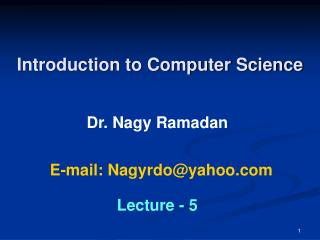DownloadDownload PresentationIntroduction to Computer Science

# Introduction to Computer Science

Download Presentation## Introduction to Computer Science

- - - - - - - - - - - - - - - - - - - - - - - - - - - E N D - - - - - - - - - - - - - - - - - - - - - - - - - - -
##### Presentation Transcript

1. Introduction to Computer Science Dr. Nagy Ramadan E-mail: Nagyrdo@yahoo.com Lecture - 5

2. Outline • Signed-magnitude system. • Signed-complement system. • Excess System. • Examples

3. The Most common notations are: • Signed-magnitude system. • Signed-complement system. • Excess System. Sign bit 0 positive Sign bit 1 negative Sign bit 1 positive Sign bit 0 negative Signed Binary Numbers It is usual to represent the sign with a bit placed in the leftmost position of the binary number. Sign bit

4. Signed-magnitude system. Ex1: 01001  + 9 11001  – 9 Ex2: 3-bit binary pattern Note: For a n-bit binary pattern, the signed-magnitude decimal range is –(2n-1-1)10, +(2n-1-1)10

5. Signed-complement system • In this system, a negative number is indicated by its complement. • Since positive numbers always start with 0 (i.e. +) in the leftmost position, the complement will always starts with 1 (i.e. -) • The signed-complement system can use either the 1’s complement or the 2’s complement notations. • Changing the sign of the binary number in the 1’s complement system is obtained by taking the 1’s complement of the binary number. • Changing the sign of the binary number in the 2’s complement system is obtained by taking the 2’s complement of the binary number.

6. EX: Assuming the representation of the number 9 in binary with 8-bits, we have the following cases: • Unsigned 9 or +9 has a the same representation in both signed-magnitude and signed-complement systems which is: 00001001 • -9 has the signed-magnitude representation: 10001001 • -9 has the signed-1’s complement representation:11110110 • -9 has the signed-2’s complement representation:11110111

7. EX The signed-complement conversion table of a 3-bit binary pattern is as follows:

8. EX: Obtain the decimal value of the binary number (11111001.101)2 in case of: • Unsigned binary notation • Signed-magnitude notation • Signed-1’s complement notation • Signed-2’s complement notation

9. Sol: • Unsigned binary notation (11111001.101)2=1*27+1*26+1*25+1*24+1*23+1*20+1*2-1+1*2-3 =128 + 64 + 32 + 16 + 8 + 1 + 0.5 + 0.125=(249.625)10 B.Signed-magnitude notation (s) (11111001.101)2= - (1*26+1*25+1*24+1*23+1*20+1*2-1+1*2-3) = - (64 + 32 + 16 + 8 + 1 + 0.5 + 0.125)= - (121.625)10

10. C. Signed-1’s complement notation - 1’s complement of (11111001.101)2 = - (0000110.010) 2 = - (6.25)10 D. Signed-2’s complement notation - 2’s complement of (11111001.101)2 = - (0000110.011) 2 = - (6.375)10

11. Excess Notation • In this system, any binary number having 1 in the leftmost bit is considered positive number. • All negative numbers have 0 in the leftmost bit.

12. EX The excess notation conversion table of a 3-bit binary pattern is as follows: • The above table is called Excess Four Conversion Table, it is obtained by subtracting 4 from the corresponding unsigned value.

13. EX: Convert each of the following excess eight notations to its equivalent decimal form: a) 1101 b) 0100 c) 0000 Sol: Excess decimal value=unsigned decimal value – 8 a) (1101)  13 – 8 = + 5 b) (0100)  4 – 8 = - 4 c) (0000)  0 – 8 = - 8

14. EX: Convert each of the following decimal values to its equivalent excess eight notations form: a) 6 b) - 6 c) 0 Sol: a) 6 + 8 = 14  (1110) b) – 6 + 8 = 2  (0010) c) 0 + 8 = 8  (1000)